Function Repository Resource:

# SignedVectorAngle

A version of VectorAngle for 2D vectors that can return negative angles

Contributed by: Jon McLoone
 ResourceFunction["SignedVectorAngle"][u,v] gives the angle between the 2D vectors u and v, returning a positive number if v is counterclockwise relative to u and negative if clockwise.

## Examples

### Basic Examples (2)

Return the angle between two vectors:

 In:=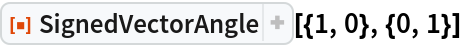Out=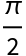If the second vector is clockwise from the first vector, the result is negative:

 In:=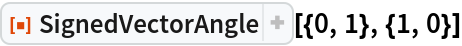Out=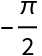### Properties and Relations (1)

The absolute value of SignedVectorAngle is always the same as VectorAngle:

 In:=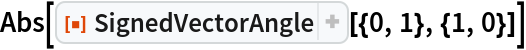Out=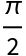In:=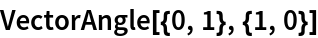Out=Jon McLoone

## Version History

• 1.0.0 – 31 May 2019